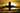Cosine Haversine formula in Rust

June 12, 2020I recently had to work with an implementation of Cosine Haversine formula in Javascript. I decided I wanted to understand how this worked in more detail, so reimplemented the algorithm in Rust to perform some tests.

Implemtation in Rust:

fn main() {
let departure_lat: f64 = 48.35;
let departure_lng: f64 = 11.79;
let arrival_lat: f64 = 51.48;
let arrival_lng: f64 = -0.46;

let result = (part_one + part_two).acos() * 6371.0;

println!("Distance in km: {:.2}", result);
}

Output:

jon@PurpleHexagon:~/code/rust/haversine\$ cargo run Compiling haversine v0.1.0 (/home/jon/code/rust/haversine) Finished dev [unoptimized + debuginfo] target(s) in 0.36s Running target/debug/haversine Distance in km: 942.20

More:

The word Haversine comes from another trigonometric function, much like the well known sine, cosine and, tangent.

This formula will calculate the great-circle distance between two points. That is the shortest distance between two points. So if you were to fly between LA and New York it would calculate the distance as if you were flying across the USA not flying all the way round the world. To use this function the latitude and longitude of the departure and arrival locations are required.

Using the formula becomes more important over longer distances where the curvature of the earth will have more impact.

Whilst this is only a rough approximation, as it does not take into account flight plans or earth’s topology, it is still very useful when calculating distances on a curved plane.